We already know that cos(z) is periodic with period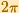.

In this page we are going to explore how an horizontal line is transformed by the function cos(z), following Tristan Needham's Visual Complex Analysis.

Playing the animation we can see that the image ofis "some kind of symmetrical oval" (is just the sum of two circular motions).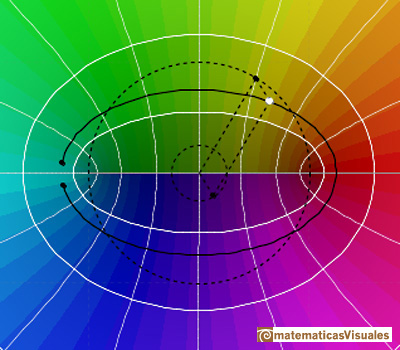To calculate where this oval hits the real axis, we consider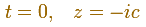Then

The oval hits the real axis at this point: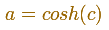To calculate where this oval hits the imaginary axis, we considerThen

The oval hits the imaginary axis at this point:The oval is a perfect ellipse. If we calculate

UsingConsidering the real and imaginary partsWe can write

Which is the familiar representation of the ellipse, that we can write (implicit formula):

To calculate the foci of the ellipse we can use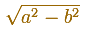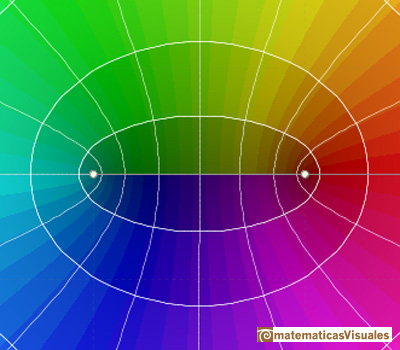The shapes of the ellipse change as we vary c but all these ellipses are confocal.

The image under cos(z) of a vertical line is an hyperbola with the same foci as the ellipse. Ellipses and hyperbolas meet at right angles.

REFERENCES

Tristan Needham - Visual Complex Analysis. (pag. 88-89) - Oxford University PressThe Complex Cosine Function extends the Real Cosine Function to the complex plane. It is a periodic function that shares several properties with his real ancestor.The power series of the Cosine Function converges everywhere in the complex plane.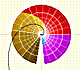The Complex Exponential Function extends the Real Exponential Function to the complex plane.By increasing the degree, Taylor polynomial approximates the sine function more and more.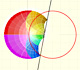Inversion is a plane transformation that transform straight lines and circles in straight lines and circles.The usual definition of a function is restrictive. We may broaden the definition of a function to allow f(z) to have many differente values for a single value of z. In this case f is called a many-valued function or a multifunction.Examples of complex functions: polynomials, rationals and Moebius Transformations.